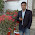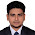## Friday, February 8, 2013

### Week-3: 3.5: For Loop

For:

As we mentioned earlier, the programming you are doing now is sequential
programming. This means that flow is downward, from top to bottom, with every line of
code being executed, unless you tell Java otherwise.

You saw in the last section that one way to "tell" Java not to execute every line is by
using IF Statement to section off areas of code.

Another way to interrupt the flow from top to bottom is by using loops. A programming
loop is one that forces the program to go back up again. If it is forced back up again
you can execute lines of code repeatedly.

As an example, suppose you wanted to add up the numbers 1 to 10. You could do it quite
easily in Java like this:

int addition = 1 + 2 + 3 + 4 + 5 + 6 + 7 + 8 + 9 + 10;

But you wouldn't really want to use that method if you needed to add up the numbers 1
to a 1000. Instead, you can use a loop to go over a line of code repeatedly until you've
reached 1000. Then you can exit the loop and continue on your way.

### Visit for Details

1./*
* To change this template, choose Tools | Templates
* and open the template in the editor.
*/
package javapractice;
import java.util.Scanner;
/**
*
* @author student
*/
public class JavaPractice {
//Scanner input=new Scanner (System.in);
/**
* @param args the command line arguments
*/

public static void main(String[] args) {
// TODO code application logic here
int a, b, sum = 0;
Scanner input=new Scanner (System.in);
// a = input.nextInt();
//b= input.nextInt();
b=5;
//sum=a+b;
for(a=1; a<b; a++)
sum=sum+a;
System.out.print("Sum is ="+sum);
System.out.print("\n");

}
}

2.ID : 201420763
packege forloop;
public class forLoop{
public static void main(String)[]args{
for(i=0; i<=90;i++){
System.out .println("This is for loop i="+i);
}
}
}

3.nice article for beginners.thank you.
javacodegeeks
welookups